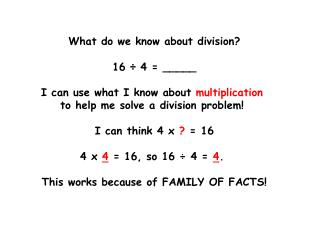DownloadDownload PresentationWhat do we know about division? 16 ÷ 4 = _____ I can use what I know about multiplication

# What do we know about division? 16 ÷ 4 = _____ I can use what I know about multiplication

Télécharger la présentation## What do we know about division? 16 ÷ 4 = _____ I can use what I know about multiplication

- - - - - - - - - - - - - - - - - - - - - - - - - - - E N D - - - - - - - - - - - - - - - - - - - - - - - - - - -
##### Presentation Transcript

1. What do we know about division? 16 ÷ 4 = _____ I can use what I know about multiplication to help me solve a division problem! I can think 4 x ? = 16 4 x 4 = 16, so 16 ÷ 4 = 4. This works because of FAMILY OF FACTS!

2. Let’s try another. 25 ÷ 5 = _____ I can use what I know about multiplication to help me solve a division problem! I can think ___ x ? = ___ ___ x ____ = ____, so 25 ÷ 5 = _____. This works because of FAMILY OF FACTS!

3. Talk this through with a partner. 36 ÷ 6 = _____ I can use what I know about multiplication to help me solve a division problem! I can think ___ x ? = ___ ___ x ____ = ____, so 36 ÷ 6 = _____. This works because of FAMILY OF FACTS!

4. So let’s review... Factor x Factor = Product Product ÷ Factor = Factor This works because of FAMILY OF FACTS! Factor Factor Product

5. We know how to use arrays to multiply! 22 x 4 4 x 20 = ? 4 x 2= ? 20 2 80 8 4 80 + 8 = 88. So 22 x 4 = 88.

6. We can also use an array to solve a division problem! 24 ÷ 4 4 x ? = 24 6 4 24 6 x 4 = 24 So 24 ÷ 4 = 6.

7. We can also use an array to solve a division problem! 84 ÷ 4 4 x ? = 80 4 x ? = 4 20 1 80 4 4 20 + 1 = 21. 4 x 21 = 84 So 84 ÷ 4 = 21.

8. We can also use an array to solve a division problem! 88 ÷ 8 8 x ? = 80 8 x ? = 8 10 1 80 8 8 10 + 1 = 11. 8 x 11 = 88 So 88 ÷ 8 = 11.

9. We can also use an array to solve a division problem! 36 ÷ 3 3 x ? = 30 3 x ? = 6 10 2 30 6 3 10 + 2 = 12 3 x 12 = 36 So 36 ÷ 3 = 12.

10. We can also use an array to solve a division problem! 66 ÷ 3 3 x ? = 60 3 x ? = 6 20 2 60 6 3 20 + 2 = 22 3 x 22 = 66 So 66 ÷ 3 = 22.

11. Now let’s use arrays to help us solve some problems on our own! 96 ÷ 3 48 ÷ 4 88 ÷ 2 63 ÷ 3

12. Let’s check our answers! 96 ÷ 3 = 32 48 ÷ 4 = 12 + + 30 2 10 2 90 6 40 8 3 4 88 ÷ 2 = 44 63 ÷ 3 = 21 + + 40 4 20 1 80 60 3 2 8 3

13. Today we’ve been thinking about division. Division is all about sharing equally. Ms. Santos owns a neighborhood grocery store. She has 56 apples to arrange in rows for her window display. She has room for 4 rows in her window. How many apples will there be in each row if she puts the same number in each row? Use color tiles to model this problem and solve it.

14. Today we’ve been thinking about division. Division is all about sharing equally. Let’s share how we thought through this problem!

15. Could we solve Ms. Santos’ problem with an array? 56 ÷ 4 4 x ? = 40 4 x ? = 16 10 4 40 16 4 10 + 4 = 14 4 x 14 = 56 So 56 ÷ 4 = 14.

16. Let’s try another problem like this. 60 ÷ 5 5 x ? = 50 5 x ? = 10 10 2 50 10 5 10 + 2 = 12 5 x 12 = 60 So 60 ÷ 5= 12.

17. Let’s try another problem like this. 72 ÷ 6 6 x ? = 60 6 x ? = 12 10 2 60 12 6 10 + 2 = 12 6 x 12 = 72 So 72 ÷ 6= 12.

18. Let’s try another problem like this. 75 ÷ 5 5 x ? = 50 5 x ? = 25 10 5 50 25 5 10 + 5 = 15 5 x 15 = 75 So 75 ÷ 5= 15.

19. Let’s try another problem like this. 90 ÷ 6 6 x ? = 60 6 x ? = 30 10 5 60 30 6 10 + 5 = 15 6 x 15 = 90 So 90 ÷ 6= 15.

20. Let’s PRACTICE! Problem: 52 ÷ 4 = ___ Problem: 64 ÷ 4 = ___ Problem: 57 ÷ 3 = ___ Problem: 66 ÷ 3 = ___